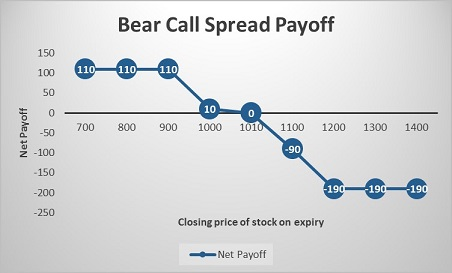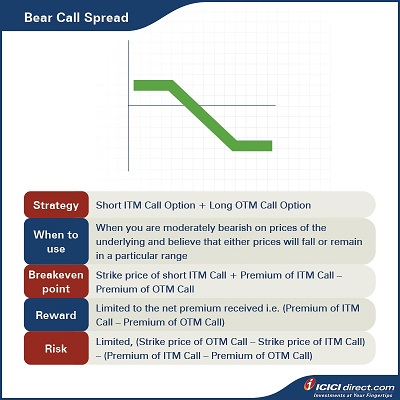Abhinav’s manager goes on to explain the Bear Call Spread to him, saying that it is a strategy that can be deployed when the investor is moderately bearish. It is also suitable for earning premium income during volatile times.

## Looking at the Bear Call Spread

In a Bear Call Spread strategy, you buy an OTM Call Option and simultaneously sell an ITM Call Option with the same expiry, on the same underlying asset and involving the same number of options. You will receive net cash inflow at the start of trade. Do note that the premium paid is less than the premium received

 Did you know?  TBear Call Spread is also known as Short Call Spread or Credit Call Spread.

This strategy can be used when you are moderately bearish on the prices of the underlying and believe that prices will either fall or remain rangebound. You protect the downside risk of the Call sold (obligation to sell the asset) by purchasing a Call of a higher strike price (right to buy the asset).

• The maximum loss that can be incurred will be equal to the difference between the two strike prices and the net premium received at the start.
• Maximum risk: Limited, (Strike price of OTM Call – Strike price of ITM Call) – (Premium of ITM Call – Premium of OTM Call).

Strategy: Short ITM Call Option (Leg 1) + Long OTM Call Option (Leg 2)

When to use: When you are moderately bearish on prices of the underlying and believe that prices will either fall or remain rangebound

Breakeven: Strike price of short ITM Call + Premium of ITM Call – Premium of OTM Call

Maximum risk: Limited, (Strike price of OTM Call – Strike price of ITM Call) – (Premium of ITM Call – Premium of OTM Call)

Let’s understand the Bear Call Spread strategy with an example:

Assume that the spot price of ABC Ltd. is Rs. 1,000. Abhinav sold an ABC Ltd. ITM Call of strike price Rs. 900 at Rs. 140 and bought an OTM Call Option of strike price Rs. 1,200 at Rs. 30. He received a total premium of Rs. 140 – Rs. 30 = Rs. 110, and this will be the maximum profit. He will start losing maximum amount if the stock moves beyond the higher strike price i.e., Rs. 1,200.

Let’s take a look at the cash flow in various scenarios:

 Closing price of stock on expiry (Rs.) Payoff from ITM Call Option (A) (Rs.) Payoff from OTM Call Option (B) (Rs.) Net payoff (A+B) (Rs.) 700 140 – 30 110 800 140 – 30 110 900 140 – 30 110 1000 40 – 30 10 1010 30 – 30 0 1100 – 60 – 30 – 90 1200 – 160 – 30 – 190 1300 – 260 70 – 190 1400 – 360 170 – 190

Let us understand the payoff in various scenarios. It will give you a fair idea of how we have arrived at the above values.

### If the stock closes at Rs. 800 on expiry: Both legs expire OTM

Leg 1: Premium received on the ITM Call Option of strike price Rs. 900 = Rs. 140

Premium paid on ITM Call Option of strike price Rs. 900 at expiry = Max {0, (Spot price – Strike price)} = Max {0, (800 – 900)} = Max (0, – 100) = 0

So, payoff from the ITM Call Option = Premium received – Premium paid = 140 – 0 = Rs. 140

Leg 2: Premium paid on the OTM Call Option of strike price Rs. 1200 = Rs. 30

Premium received on OTM Call Option of strike price Rs. 1200 at expiry = Max {0, (Spot price – Strike price)} = Max {0, (800 – 1200)} = Max (0, – 400) = 0

So, the payoff from the OTM Call Option = Premium received – Premium paid = 0 – 30 = – Rs. 30

Net payoff = Payoff from ITM Call Option + Payoff from OTM Call Option = 140 – 30 = Rs. 110

### If the stock closes at Rs. 1010 on expiry: Leg 1 expires ITM while leg 2 expires OTM

Leg 1: Premium received on the ITM Call Option of strike price Rs. 900 = Rs. 140

Premium paid on ITM Call Option of strike price Rs. 900 at expiry = Max {0, (Spot price – Strike price)} = Max {0, (1010 – 900)} = Max (0, 110) = Rs. 110

So, the payoff from the ITM Call Option = Premium received – Premium paid = 140 – 110 = Rs. 30

Leg 2: Premium paid on the OTM Call Option of strike price Rs.1200 = Rs. 30

Premium received on the OTM Call Option of strike price Rs. 1200 at expiry = Max {0, (Spot price – Strike price)} = Max {0, (1010 – 1200)} = Max (0, – 190) = 0

So, the payoff from the OTM Call Option = Premium received – Premium paid = 0 – 30 = – Rs. 30

Net payoff = Payoff from ITM Call Option + Payoff from OTM Call Option = 30 + (– 30) = 0

### lf the stock closes at Rs. 1300 on expiry: Both legs expire ITM

Leg 1: Premium received on the ITM Call Option of strike price Rs. 900 = Rs. 140

Premium paid on the ITM Call Option of strike price Rs. 900 at expiry = Max {0, (Spot price – Strike price)} = Max {0, (1300 – 900)} = Max (0, 400) = Rs. 400

So, the payoff from the ITM Call Option = Premium received – Premium paid = 140 – 400 = – Rs. 260

Leg 2: Premium paid on the OTM Call Option of strike price Rs.1200 = Rs. 30

Premium received on OTM Call Option of strike price Rs. 1200 at expiry = Max {0, (Spot price – Strike price)} = Max {0, (1300 – 1200)} = Max (0, 100) = Rs.100

So, the payoff from the OTM Call Option = Premium received – Premium paid = 100 – 30 = Rs. 70

Net payoff = Payoff from ITM Call Option + Payoff from OTM Call Option = (– 260) + 70 = – Rs. 190## Summary